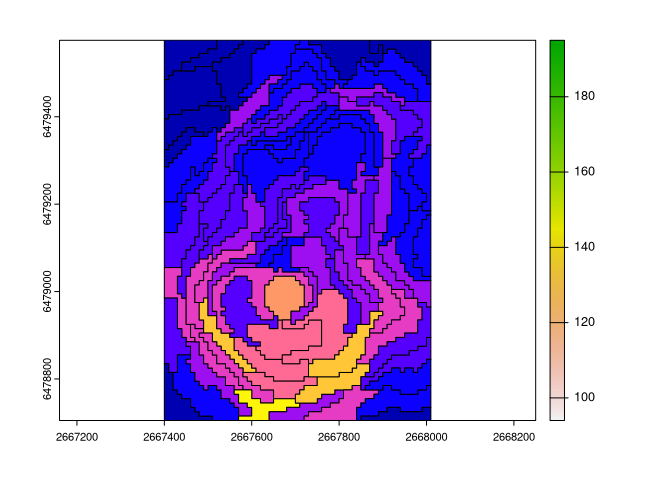# regional

The regional package calculates intra-regional and inter-regional similarities based on user-provided spatial vector objects (regions) and spatial raster objects (cells with values). Implemented metrics include inhomogeneity, isolation (Haralick and Shapiro (1985), https://doi.org/10.1016/S0734-189X(85)90153-7>, Jasiewicz et al. (2018), https://doi.org/10.1016/j.cageo.2018.06.003), and distinction (Nowosad (2021), https://doi.org/10.1080/13658816.2021.1893324).

## Installation

You can install the released version of regional from CRAN with:

``install.packages("regional")``

You can install the development version from GitHub with:

``````# install.packages("remotes")

## Example

``````library(regional)
library(terra)
library(sf)
volcano = rast(system.file("raster/volcano.tif", package = "regional"))
vr = read_sf(system.file("regions/volcano_regions.gpkg", package = "regional"))
plot(volcano)### Inhomogeneity

How much internally inconsistent each region is?

``````vr\$inh = reg_inhomogeneity(vr, volcano, sample_size = 0.5)
plot(volcano)``````mean(vr\$inh)
#>  2.746237``````

### Isolation

How much the focus region differs from its neighbors?

``````vr\$iso = reg_isolation(vr, volcano, sample_size = 1)
plot(volcano)``````mean(vr\$iso)